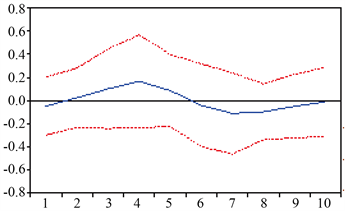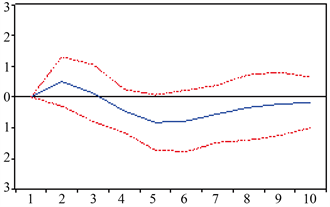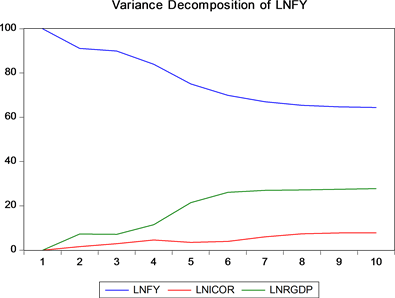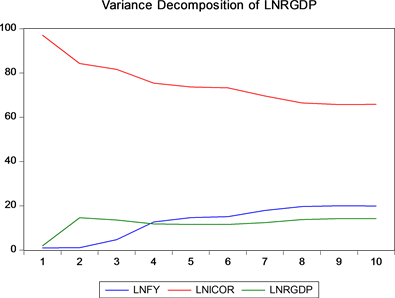﻿ 河南省空气污染与经济增长关系实证研究

# 河南省空气污染与经济增长关系实证研究An Empirical Study on the Relationship between Air Pollution and Economic Growth in Henan Province

Abstract: The VAR model was used to empirically study the dynamic relationship between economic growth and air pollution in Henan Province. The empirical results show that the elasticity of GDP for SO2 emissions in the period from 2000 to 2015 is 0.164. The elasticity of smoke (powder) dust emissions is −0.105. There is a long-term and stable relationship between economic growth and air pollution in Henan Province. The relationship between economic growth and air pollution presents an “inverted U-shaped” and “N-shaped” relationship, which is different from other provinces and cities; air pollution affects economic growth. The negative impact will only appear after a long lag period, in which the negative impact of SO2 on economic growth will lag for about 5 years, and the negative impact of smoke (powder) dust on economic growth will lag for about 3 years; The contribution of SO2 emissions quantity to the forecast variance of economic growth is greater than the contribution of smoke (powder) dust to the forecast variance of economic growth.

1. 引言

2. 模型的设定及指标说明

${Y}_{t}={\alpha }_{1}{Y}_{t-1}+{\alpha }_{2}{Y}_{t-2}+\cdots +{\alpha }_{p}{Y}_{t-p}+{\epsilon }_{t}$

3. 实证检验与分析

3.1. 建立VAR模型Table 1. Selection and Explanation of Variables Related to Economic Growth and Air Pollution in Henan ProvinceTable 2. The choice of lag periodFigure 1. Root diagram of the AR

3.2. 脉冲响应分析

3.3. 方差分解Figure 2. Impulse response of economic growth to smoke dust emissionFigure 3. Impulse response of smoke emission to economic growthFigure 4. Variance decomposition of smoke (powder) dust emissions (Unit: %)Figure 5. Variance of economic growth decomposition (Unit: %)

4. 结论及建议

${\text{SO}}_{2}$ 排放量对经济增长的预测方差的贡献要大于烟(粉)尘对经济增长的预测方差的贡献。说明 排放量对经济增长的抑制作用要大于烟(粉)尘，虽然当前河南省主要大气污染物是PM2.5，大气污染治理有治理烟(粉)尘污染为主，但是以 ${\text{SO}}_{2}$ 为主要排放物的各种大气污染依然不能忽视，空气污染治理需全面、综合治理。

 李娟伟, 任保平. 协调中国环境污染与经济增长冲突的路径研究——基于环境退化成本的分析[J]. 中国人口•资源与环境, 2015(5): 132-139.

 李小胜, 张焕明. 中国经济增长、污染排放与能源消费间动态关系研究——基于面板VAR模型的实证[J]. 山西财经大学学报, 2013(11): 25-34.

 李治国, 周德田. 基于VAR模型的经济增长与环境污染关系实证分析——以山东省为例[J]. 企业经济, 2013(8): 11-16.

Top